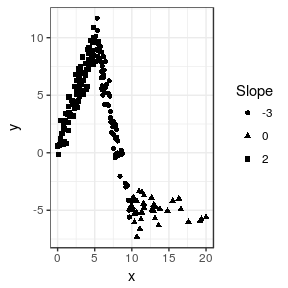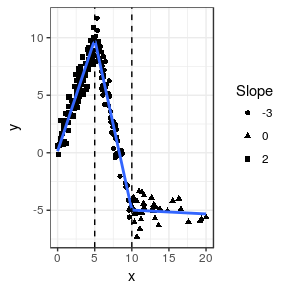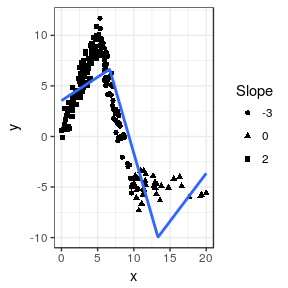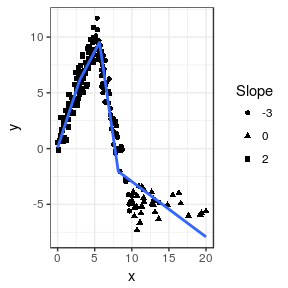lspline: Linear Splines with Convenient Parametrisations

Linear splines with convenient parametrisations such that

• coefficients are slopes of consecutive segments
• coefficients capture slope change at consecutive knots

Knot locations can be specified

• manually (lspline())
• at breaks dividing the range of x into q equal-frequency intervals (qlspline())
• at breaks dividing the range of x into n equal-width intervals (elspline())

Examples

Examples of using lspline(), qlspline(), and elspline(). We will use the following artificial data with knots at x=5 and x=10

set.seed(666)
n <- 200
d <- data.frame(
x = scales::rescale(rchisq(n, 6), c(0, 20))
)
d$interval <- findInterval(d$x, c(5, 10), rightmost.closed = TRUE) + 1
d$slope <- c(2, -3, 0)[d$interval]
d$intercept <- c(0, 25, -5)[d$interval]
d\$y <- with(d, intercept + slope * x + rnorm(n, 0, 1))

Plotting y against x:

library(ggplot2)
fig <- ggplot(d, aes(x=x, y=y)) +
geom_point(aes(shape=as.character(slope))) +
scale_shape_discrete(name="Slope") +
theme_bw()
figThe slopes of the consecutive segments are 2, -3, and 0.

Setting knot locations manually

We can parametrize the spline with slopes of individual segments (default marginal=FALSE):

library(lspline)
m1 <- lm(y ~ lspline(x, c(5, 10)), data=d)
knitr::kable(broom::tidy(m1))
term estimate std.error statistic p.value
(Intercept) 0.1343204 0.2148116 0.6252941 0.5325054
lspline(x, c(5, 10))1 1.9435458 0.0597698 32.5171747 0.0000000
lspline(x, c(5, 10))2 -2.9666750 0.0503967 -58.8664832 0.0000000
lspline(x, c(5, 10))3 -0.0335289 0.0518601 -0.6465255 0.5186955

Or parametrize with coeficients measuring change in slope (with marginal=TRUE):

m2 <- lm(y ~ lspline(x, c(5,10), marginal=TRUE), data=d)
knitr::kable(broom::tidy(m2))
term estimate std.error statistic p.value
(Intercept) 0.1343204 0.2148116 0.6252941 0.5325054
lspline(x, c(5, 10), marginal = TRUE)1 1.9435458 0.0597698 32.5171747 0.0000000
lspline(x, c(5, 10), marginal = TRUE)2 -4.9102208 0.0975908 -50.3143597 0.0000000
lspline(x, c(5, 10), marginal = TRUE)3 2.9331462 0.0885445 33.1262479 0.0000000

The coefficients are

• lspline(x, c(5, 10), marginal = TRUE)1 - the slope of the first segment
• lspline(x, c(5, 10), marginal = TRUE)2 - the change in slope at knot $$x=5$$; it is changing from 2 to -3, so by -5
• lspline(x, c(5, 10), marginal = TRUE)3 - tha change in slope at knot $$x=10$$; it is changing from -3 to 0, so by 3

The two parametrisations (obviously) give identical predicted values:

all.equal( fitted(m1), fitted(m2) )
##  TRUE

graphically

fig +
geom_smooth(method="lm", formula=formula(m1), se=FALSE) +
geom_vline(xintercept = c(5, 10), linetype=2)Knots at n equal-length intervals

Function elspline() sets the knots at points dividing the range of x into n equal length intervals.

m3 <- lm(y ~ elspline(x, 3), data=d)
knitr::kable(broom::tidy(m3))
term estimate std.error statistic p.value
(Intercept) 3.5484817 0.4603827 7.707678 0.00e+00
elspline(x, 3)1 0.4652507 0.1010200 4.605529 7.40e-06
elspline(x, 3)2 -2.4908385 0.1167867 -21.328105 0.00e+00
elspline(x, 3)3 0.9475630 0.2328691 4.069080 6.84e-05

Graphically

fig +
geom_smooth(aes(group=1), method="lm", formula=formula(m3), se=FALSE, n=200)Knots at quantiles of x

Function qlspline() sets the knots at points dividing the range of x into q equal-frequency intervals.

m4 <- lm(y ~ qlspline(x, 4), data=d)
knitr::kable(broom::tidy(m4))
term estimate std.error statistic p.value
(Intercept) 0.0782285 0.3948061 0.198144 0.8431388
qlspline(x, 4)1 2.0398804 0.1802724 11.315548 0.0000000
qlspline(x, 4)2 1.2675186 0.1471270 8.615132 0.0000000
qlspline(x, 4)3 -4.5846478 0.1476810 -31.044273 0.0000000
qlspline(x, 4)4 -0.4965858 0.0572115 -8.679818 0.0000000

Graphically

fig +
geom_smooth(method="lm", formula=formula(m4), se=FALSE, n=200)Installation

Stable version from CRAN or development version from GitHub with

devtools::install_github("mbojan/lspline", build_vignettes=TRUE)

Acknowledgements

Inspired by Stata command mkspline and function ares::lspline from Junger & Ponce de Leon (2011). As such, the implementation follows Greene (2003), chapter 7.5.2.

• Greene, William H. (2003) Econometric analysis. Pearson Education
• Junger & Ponce de Leon (2011) ares: Environment air pollution epidemiology: a library for timeseries analysis. R package version 0.7.2 retrieved from CRAN archives.